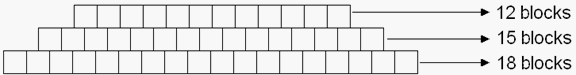Skill 24J Visual Patterns We've previously covered the skill of identifying patterns using just numbers. Here, we introduce visual patterns that add increased complexity. The student here is asked to say how many blocks would be in the next row on top (which would be 9). We then ask the student to explain the rule for the pattern. So for this problem, the rule would be "subtract 3 for each new row on top." John is making a triangle shape out of blocks. The bottom row has 18 blocks. The second row has 15 blocks. The third row has 12 blocks.1. How many blocks will the fifth row have if this pattern is continued? 2.  What is the rule for solving this problem?